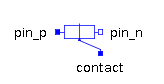Chopper Step Up - MapleSim Help

Chopper Step Up

Step-up chopperDescription The Chopper Step Up component is a conventional step-up chopper (boost converter) model. It consists of a transistor and free wheeling diode. The gate of the pass-transistor is controlled by the boolean input ${\mathrm{fire}}_{p}$ and enabled with either a parameter or an enable input.Connections

 Name Description Modelica ID ${\mathrm{dc}}_{\mathrm{p1}}$ Electrical port; positive DC input dc_p1 ${\mathrm{dc}}_{\mathrm{n1}}$ Electrical port; negative DC input dc_n1 ${\mathrm{dc}}_{\mathrm{p2}}$ Electrical port; positive DC output dc_p2 ${\mathrm{dc}}_{\mathrm{n2}}$ Electrical port; negative DC output dc_n2 $\mathrm{heatPort}$ heatPort $\mathrm{enable}$ Boolean input; enables fire and notFire enable ${\mathrm{fire}}_{p}$ Boolean input; firing signal of transistor fire_pParametersGeneral Parameters

 Name Default Units Description Modelica ID ${R}_{\mathrm{on}\left(\mathrm{tran}\right)}$ $1·{10}^{-5}$ $\mathrm{\Omega }$ Transistor closed resistance RonTransistor ${G}_{\mathrm{off}\left(\mathrm{tran}\right)}$ $1·{10}^{-5}$ $S$ Transistor opened conductance GoffTransistor ${V}_{\mathrm{knee}\left(\mathrm{tran}\right)}$ $0$ $V$ Transistor threshold voltage VkneeTransistor ${R}_{\mathrm{on}\left(\mathrm{diode}\right)}$ $1·{10}^{-5}$ $\mathrm{\Omega }$ Closed diode resistance RonDiode ${G}_{\mathrm{off}\left(\mathrm{diode}\right)}$ $1·{10}^{-5}$ $S$ Opened diode conductance GoffDiode ${V}_{\mathrm{knee}\left(\mathrm{diode}\right)}$ $0$ $V$ Diode forward threshold voltage VkneeDiode Use Heat Port $\mathrm{false}$ True (checked) means heat port is enabled useHeatPortEnable Parameters

 Name Default Units Description Modelica ID Use Constant Enable $\mathrm{true}$ True (checked) means disable Enable port. useConstantEnable Constant Enable $\mathrm{true}$ True (checked) means firing signals are always enabled. constantEnableModelica Standard Library The component described in this topic is from the Modelica Standard Library. To view the original documentation, which includes author and copyright information, click here.